# Forward Cover Functionality## Summary Statement

In the supply chain horizontal, you may be asked to calculate a forward cover in Anaplan. In this article, we modified a best practice suggestion from @nathan_rudman based on a question posed by @mikhivin about accounts receivable coverage which looks backward. See this post on count back for turnover. The forward weeks of cover example uses ending inventory as the basis and asks the question, "How many days or weeks will it take to sell through the inventory?" A product hierarchy is also added to show how easily this method can be used across dimensions.

## Forward Cover Functionality

Unfortunately, a forward cover function doesn't exist yet in Anaplan. @nathan_rudman devised a process that will simulate a backward-looking function. The steps to accomplish a forward-looking solution is shown in this modified approach. The steps to accomplish this are as follows:

1. Determine how many months it will take to exhaust the inventory.
2. Calculate the Forward Cover.
• Use the MOVINGSUM function to add up the sales forecasts and the number of days.
• Obtain the statistics in the last month, and bring them forward to the same month as the ending inventory being evaluated.
• Determine the sales percentage over, and use that ratio against the number of days.
• Calculate the forward cover.

Note: this example also uses the D.I.S.C.O. methodology developed by @DavidSmith.

Results

Here is what the final output will look like.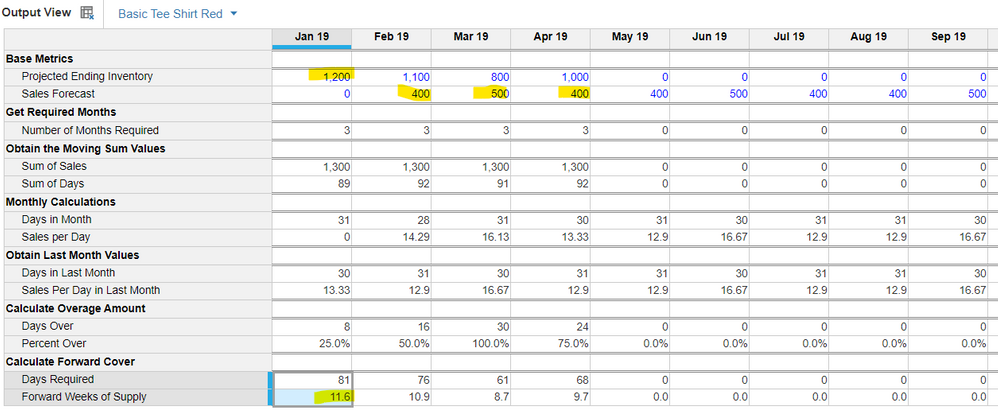Step 1: Determine the number of months to exhaust the inventory.

This is the hardest part of the process but can be accomplished rather quickly with two line items and one very small list.

Create a list containing the number of months you want to look forward. In this example, I have chosen 12 months as a maximum forward look. We will need this list because every month will have to be evaluated to determine the one that finally exhausts the inventory. Make sure to add a top value as this is necessary to find the one month we need. Mine is called All Possibilities.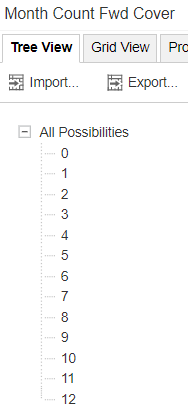The formulas in the calculation module are very intense, so it's critical to follow the D.I.S.C.O. methodology and create a system module for the Month Count Fwd Cover List you created above. The system module should contain two line items, one for the item and one for the value of that item. The value item will be calculated once in this system module, then reused in the calculation module in the next step. The blueprint view looks like this: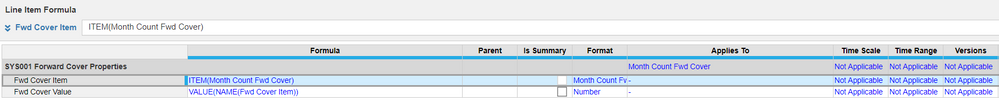Blueprint view

The normal view looks like this: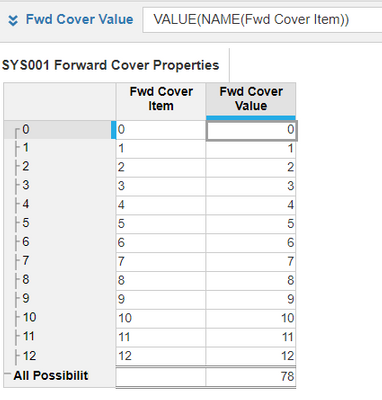Normal view

Next, create a calculation module using this list and the time dimension. I called mine CAL001 How Many Months. In this example, I am using months, but it works the same with weeks. You'll just need to modify your list to be weeks forward instead of months. Add two line items that reference the sales and inventory. Months needed simply tests every month to determine if we've exceeded the ending inventory. If we have, then record the month number. If not, then use the ending inventory so we exclude that month when we summarize this line item. The Months needed should have a "Min' summary. The Min summary will take the lowest number, so using ending inventory will ensure we don't include it. If ending inventory is zero, then our forward cover will be zero. If statements should be designed to exit as early as possible, so the most likely outcome should be first.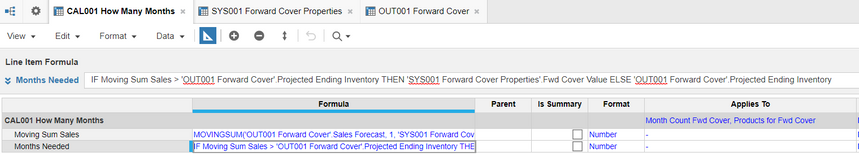• Moving Sum Sales | MOVINGSUM('OUT001 Forward Cover'.Sales Forecast, 1, 'SYS001 Forward Cover Properties'.Fwd Cover Value)
• Months Needed | IF Moving Sum Sales > 'OUT001 Forward Cover'.Ending Inventory THEN 'SYS001 Forward Cover Properties'.Fwd Cover Value ELSE 'OUT001 Forward Cover'.Ending Inventory

Notice how the summary contains the correct number of months needed to exhaust the inventory.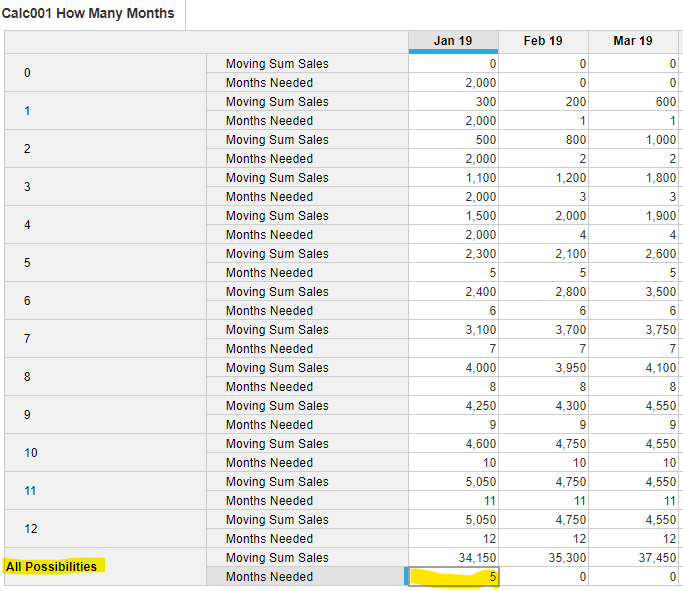## Step 2: Calculate the Forward Cover.

The last step is straight-forward since we now know how many months it will take. Create the following line items to your output module. Mine is called OUT001 Forward Cover. You should also create an SYS002 Time Properties Module as well that includes one line item needed to calculate Days In Month by using the DAYS() function. This will prevent the OUT001 module from calculating this unnecessarily.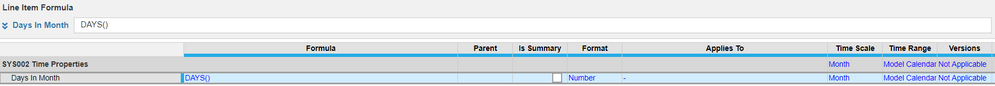Some of these line items can be combined, but I broke them up to illustrate the process which supports the PLANS methodology.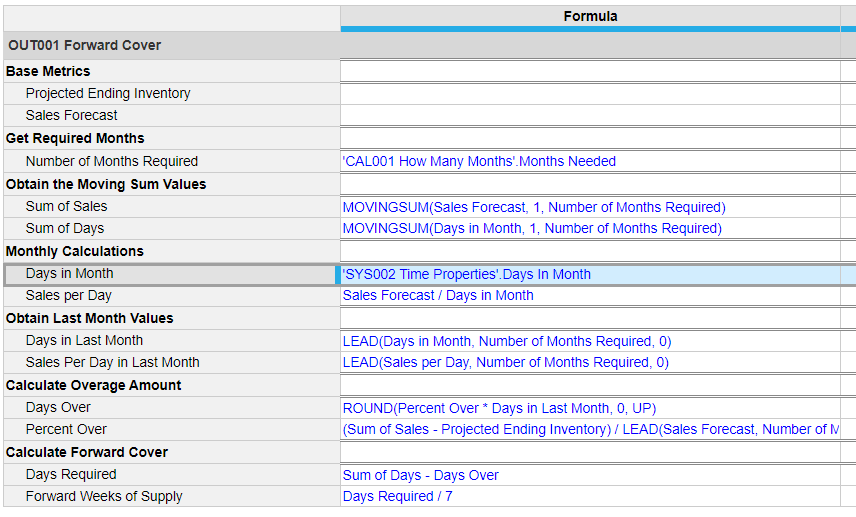Percent Over = (Sum of Sales - Projected Ending Inventory) / LEAD(Sales Forecast, Number of Months Required, 0).

And finally, you can see the results from the top of this article.

## Conclusions

I'll repeat a lot of what @nathan_rudman had to say because there are some pros/cons of this method.

• Limiting the number of months or the other dimensions—like product and location—will significantly help with performance since this is a rather intensive calculation testing every possible combination.
• Limit how far you will look forward. You can even start at month two or three if you think anything less than eight weeks of supply is not worth analyzing.
• Utilize system modules to filter your data (see D.I.S.C.O.).
• Finally, impress your supply chain colleagues with this amazing statistic that can be created in Anaplan!

Again, I want to thank @nathan_rudman for sharing his wisdom and creativity in solving the backward-looking solution. It made creating this article much easier. Also, big thanks to @DavidSmith and @rob_marshall for reviewing this article and providing feedback.

Tagged:

•